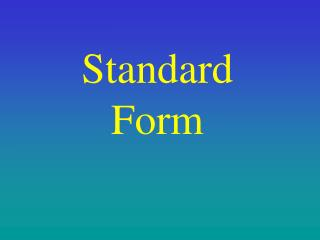DownloadDownload PresentationStandard Form

# Standard Form

Download Presentation## Standard Form

- - - - - - - - - - - - - - - - - - - - - - - - - - - E N D - - - - - - - - - - - - - - - - - - - - - - - - - - -
##### Presentation Transcript

1. Standard Form

2. 2 is multiplied by itself 3 times Revision Powers: On calculator: Try these: 65 54 36 25 94 104 10 000 Answers: 7776 625 729 32 6561

3. 3 zeros Powers of 10 Powers of 10 are easy e.g.103 = 10 x 10 x 10=1000 Look at these sequences Divide by 10 From this we see that

4. Working with big numbers Scientists often have to work with big numbers. Example: The distance the earth travels in one orbit is 558 000 000 miles. We can express this number in a more concise form as follows. 558 000 000 = 5.58 x 100 000 000 = 5.58 x 108 is said to be in Standard Form 5.58 x 108 or Scientific Notation is said to be in Normal Form 558 000 000

5. the power is a positive or negative whole number exactly one digit before the point watch this 5 jumps 63. x 105 630. 6300. 630000. 63000. 2 1 3 4 5 Look at this number which is in Standard Form 6.3 x 105 6.3 x 105 = 630 000 = 6.3 x 10 x 10 x 10 x 10 x 10 Standard form Ordinary Number 6.3

6. Example: Write 2.45 x 104 as an ordinary number. • Write the question • List the digits without the point • 4 jumps from position of “old” point • Write the answer Method: Add zeros as required 0 0 0 0 2 4 5 1 3 4 2 Now try these: Write as ordinary numbers: 4 x 103 4.88 x 101 1.47 x 102 9.08 x 106 1.3 x 100 147 9080000 1.3 4000 48.8

7. 4 1 5 3 2 watch this 5 jumps Therefore 630 000 = 6.3 x 105 x 105 Ordinary Number Standard form 630 000 = 6.3 x 100 000 = 6.3 x 105 63000. 630000. 0 0 0 6300. 0 0 0 0 0 0 0 0 0 0 0 630. 6.3 63.

8. Make the number “look” like standard form Positive power since large number Put in a decimal point to make the number “look” like a number between 1 and 10. = 2 7 0 6 Example: Write 2706 in standard form. Method: • Write the number • Insert “new” decimal point • Count jumps to position of “old” decimal point 2 7 0 6 3 2 1 x 3 10 Now try these: Write in standard form: 34560 1023.6 12.8 4.6 230000 3.456 x 104 1.0236 x 103 1.28 x 101 4.6 x 100 2.3 x 105

9. the power is a positive or negative whole number exactly one digit before the point watch this 3 jumps x 10-3 1 2 3 Numbers less than 1 Consider the number 2.03 x 10-3 This number is in standard form but is different from previous examples. Look again at powers of 10 Notice the power is negative = 0.00203 2.03 x 10-3 = 2.03 x 0.001 Standard form Ordinary Number x 10-3 2.03 .203 .0203 .00203 = 0.00203

10. Example: Write 1.07 x 10-4 as an ordinary number. • Write the question • List the digits without the point • 4 jumps from position of “old” point • Write the answer Method: Add zeros as required That means the answer starts with 0.----- Note: When the power is negative the ordinary number is always less than 1. 0 0 0 1 3 4 2 0 1 0 7 0 0 0 Now try these: Write as ordinary numbers: 4.081 x 10-4 4 x 10-3 1.47 x 10-2 9.08 x 10-5 1.3 x 10-1 0.0147 0.0000908 0.13 0.004 0.0004081

11. 1 3 2 watch this 3 jumps Therefore 630 000 = 6.31 x 10-3 x 10-3 Ordinary Number Standard form 0.00631 = 6.31 x 0.001 = 6.31 x 10-3 0.00631 0 0 0 0.631 0.0631 0 0 0 6.31

12. Make the number “look” like standard form 0 0 0 2 7 Negative power since tiny number Put in a decimal point to make the number “look” like a number between 1 and 10. = 2 7 Example: Write 0.0027 in standard form. Method: • Write the number • Insert “new” decimal point • Count jumps to position of “old” decimal point 3 2 1 3 x 10 - Now try these: Write in standard form: 0.3456 0.00102 0.0128 0.000046 0.0000000023 3.456 x 10-1 1.02 x 10-3 1.28 x 10-2 4.6 x 10-5 2.3 x 10-9

13. We use EXP button as shown on the calculator to enter numbers already in standard form. 2 6 EXP 8 = check this is correct Too large to convert 3 5 EXP 1 2 = Standard Form on the Calculator Examples: 2.6 x 108 260 000 000 3.5 x 1012 Calculator’s way of writing 3.5 x 1012 – does not mean 3.5 to the power 12! 3.5 12

14. calculator display calculator display (2.484 x 107) (4.6 x 10-4) • Only enter EXP before power, never x 10 EXP • For negative powers use the ( - ) key not the key, we are not subtracting. Calculations Giving your Answer in Standard Form Example 1: (2.6 x 108) x (4.2 x 106) = 1.092 x 1015 1.092 15 Example 2: = 5.4 x 1010 5.4 10 Note: See calculators again • Remember to change number on display to ---- x 10-- Click button to end presentation

15. ( - )

16. END